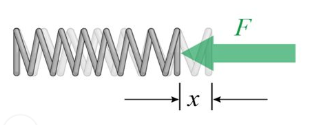# Spring Constant Formula

## Spring Constant Formulas Using Hooke's Law

It’s easy to recollect Hooke’s law in connection with spring constant. As per this law, the force required to compress or extend a spring is directly proportional to the distance it is compressed or stretched.

Mathematically, $F \propto x$, where F is the force applied, and x is the extension or compression of the object (usually in metres).Now, F = – kx, where k is the constant of proportionality called the spring constant.

(Negative sign is because, this F is actually taken to be the restoring force and the displacement happens against this force, or work is done against the restoring force. Within elastic limit, the restoring force will be equal in magnitude to the applied force)

Now; $k = --\frac{F}{x}$

Spring constant can be defined as the force required per unit extension or unit compression of the spring. Knowing the spring constant, one can easily find how much force is needed to deform the spring by certain extent (x).

Its unit is newton per meter, (i.e., N/m)

Example 1:

A spring suspended vertically and loaded at the bottom by a mass of 12 kg, extends it by 8 cm. Find the spring constant.

Solution: m = 12 kg, x = 8 cm = 8 × 10–2 m, k = ? When weight is suspended from the spring, force acting on it: F = mg = 12 × 9.8 N

Now, $k = --\frac{F}{x} = --\frac{{12 \times 9.8}}{{8 \times {{10}^{--2}}}} = --1.47 \times {10^3}N/m$

Example 2:

If the spring constant of a spring is –50 N/m, how much will it get stretched if it is fixed at one end and the other end is pulled by a force of 250 N?

Solution:

k = – 50 N/m, F = 250 N, x = ?
$F = --kx\,\,\, \Rightarrow \,\,x = \frac{F}{{--k}} = \frac{{250}}{{50}} = 5\,m$.
That is, the spring will get stretched by 5 m.

Practice question:

If the same load causes extensions of 3 cm and 8 cm in two different springs A and B respectively, then the ratio of the forces (FA : FB) required to cause the same extensions in these springs will be:
(a) 3 : 8 (b) 8 : 3 (c) 5 : 11 (d) 11 : 3

Ans (b)